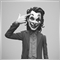# Problem with "Ordersend" function on my Expert10

Hi,

I coded a simple expert that open an order (BUYLIMIT or SELLLIMIT) when the price reach a certain level that define, I run it monday and it worked perfectly, But today I run it and it doesn't work despite that I didn't change nothing in the code, and I try it on many brokers but the problem remain, Following you will find the code and Thanks for any help

```extern double lots=0.1;
extern int stop=5;
extern int limite=10;
extern double R1=1.2255 ;
extern double S1=1.2055 ;
extern int T = 0;
extern int V= 0;
extern int magic = 3206;
//+------------------------------------------------------------------+
//| Expert initialization function                                   |
//+------------------------------------------------------------------+
int init()
{

T == 1;

return(0);

}
//+------------------------------------------------------------------+
//| Expert deinitialization function                                 |
//+------------------------------------------------------------------+
int deinit()
{
//---

//---
return(0);
}
//+------------------------------------------------------------------+
//| Expert start function                                             |
//+------------------------------------------------------------------+
int start()
{
//---
Affichage("NiveauR1","NiveauS1");

if (R1 - Ask <= 100*Point && T==0)
{

T++;
for (int i = OrdersTotal(); i >=0; i--)
{
{
if (OrderSymbol()==Symbol())
{
if (OrderType()==OP_SELL && OrderMagicNumber()==magic)
{

if ( Bid >= R1-5*Point)
{
OrderModify(OrderTicket(),OrderOpenPrice(),OrderOpenPrice(),OrderTakeProfit(),0,CLR_NONE);

}
}
}
}
}
}

//ACHAT

if (Ask - S1 <= 100*Point && T==0)
{

T++;
for (int i = OrdersTotal(); i >=0; i--)
{
{
if (OrderSymbol()==Symbol())
{
{

if ( Bid >= S1+5*Point)
{
OrderModify(OrderTicket(),OrderOpenPrice(),OrderOpenPrice(),OrderTakeProfit(),0,CLR_NONE);

}
}
}
}
}
}

//---
return(0);
}
//+------------------------------------------------------------------+
void Affichage(string NiveauR1, string NiveauS1)
{
ObjectCreate(NiveauR1,OBJ_HLINE,0,0,R1,0,0,0,0);
ObjectCreate(NiveauS1,OBJ_HLINE,0,0,S1,0,0,0,0);
}

```16398

Thabet77:

Hi,

But today I run it and it doesn't work

what is error return code?

simplify your code, and try not to use hard-coding practice.